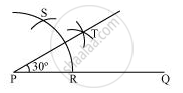Share

# Construct the angles of the following measurement:- 30° - CBSE Class 9 - Mathematics

ConceptBasic Constructions

#### Question

Construct the angles of the following measurement:- 30°

#### Solution

The below given steps will be followed to construct an angle of 30°.

Step I: Draw the given ray PQ. Taking P as centre and with some radius, draw an arc of a circle which intersects PQ at R.

Step II: Taking R as centre and with the same radius as before, draw an arc intersecting the previously drawn arc at point S.

Step III: Taking R and S as centre and with radius more than 1/2RS, draw arcs to intersect each other at T. Join PT which is the required ray making 30° with the given ray PQ.Is there an error in this question or solution?

#### APPEARS IN

NCERT Solution for Mathematics Class 9 (2018 to Current)
Chapter 11: Constructions
Ex. 11.10 | Q: 3.1 | Page no. 191

#### Video TutorialsVIEW ALL 

Solution Construct the angles of the following measurement:- 30° Concept: Basic Constructions.
S